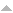# Halcyon Calc Invertible Operations

The following table contains the list of operations which have an inverse function. The ISOL operation uses these inverse functions to isolate a symbol in an expression.

OperationDescription
+

The add operation will take its two numerical operands and produce the sum as its result. It operates on reals,...

-

The subtract operation will take its two numerical operands and produce the difference as its result. It operates...

=

This operation always produces an expression which describes a relationship between its left and right side. Unlike...

ACOS

This operation calculates the inverse cosine...

ACOSH

This operation calculates the inverse hyperbolic cosine...

ALOG

This operation calculates the reverse base 10 logarithm of its...

ASIN

This operation calculates the inverse sine...

ASINH

This operation calculates the inverse hyperbolic sine...

ATAN

This operation calculates the inverse tangent...

ATANH

This operation calculates the hyperbolic sine...

CONJ

This function takes a complex value and returns its conjugate value. A conjugate value has the same real component...

COS

This operation calculates the cosine function of...

COSH

This operation calculates the hyperbolic cosine...

EXP

This operation calculates the reverse base e logarithm...

EXPM

This operation calculates the equivalent of (EXP(x) - 1) where x is the the argument...

INV

This operation takes a real of complex value and produces its inverse. The inverse is one divided by the input...

LN

This operation calculates the natural logarithm (base e)...

LNP1

This operation calculates the equivalent of LN(1 + x) where x is the the argument from...

LOG

This operation calculates the base 10 logarithm of its input...

NEG

Given a real or complex input, this function returns the value of that input multiplied by -1. Given a matrix or...

SIN

This operation calculates the sine function of...

SINH

This operation calculates the hyperbolic sine...

SQ

The SQ operation multiplies its input argument by itself to produce the square of that input value. The value can...

TAN

This operation calculates the tangent function of...

TANH

This operation calculates the hyperbolic tangent...

^

This operation calculates xy given that values x and y are pushed onto the stack in that order. Either...

×

The multiply operation will take its two numerical operands and produce the product as its result. It operates on...

÷

The divide operation will take its two numerical operands and produce the quotient as its result. It operates on...

This operation takes a real or complex value and finds its square root. The positive root is return for real values...